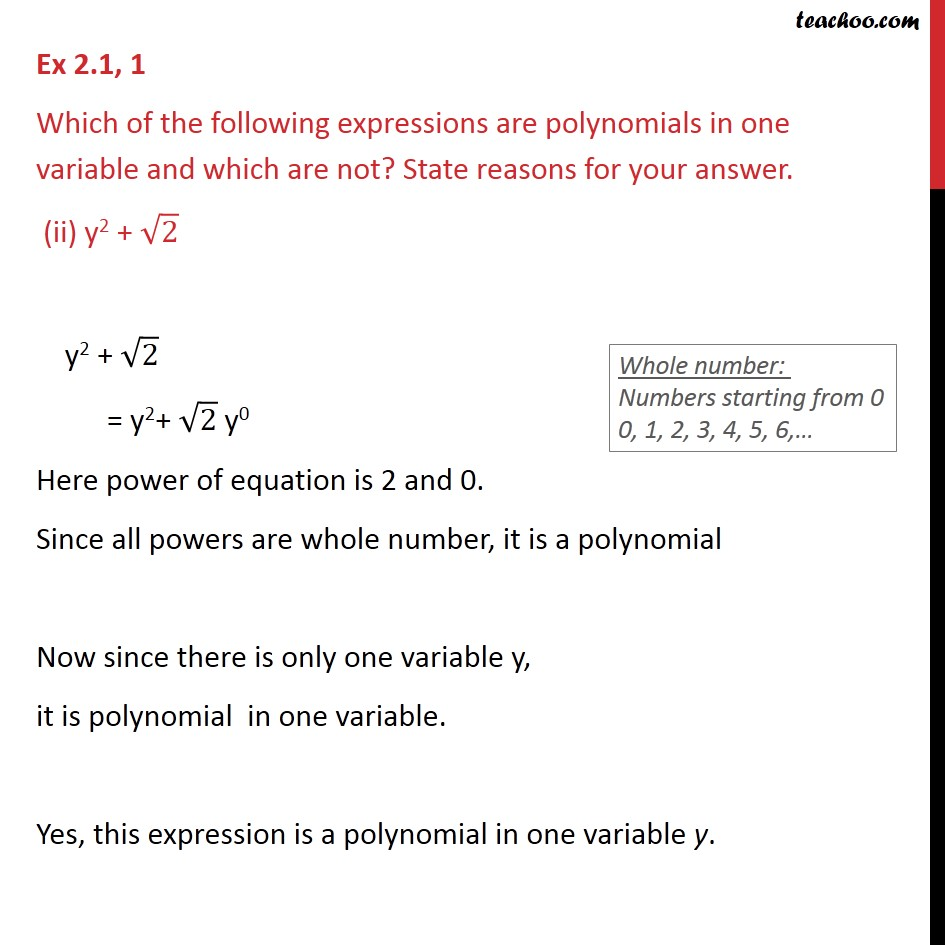Ex 2.1

Chapter 2 Class 9 Polynomials
Serial order wiseGet live Maths 1-on-1 Classs - Class 6 to 12

### Transcript

Ex 2.1, 1 Which of the following expressions are polynomials in one variable and which are not? State reasons for your answer. (ii) y2 + √2 y2 + √2 = y2+ √2 y0 Here power of equation is 2 and 0. Since all powers are whole number, it is a polynomial Now since there is only one variable y, it is polynomial in one variable. Yes, this expression is a polynomial in one variable y.